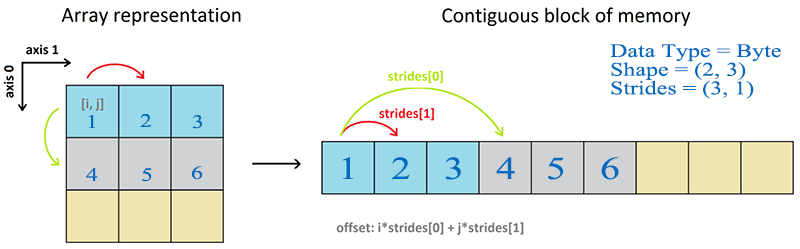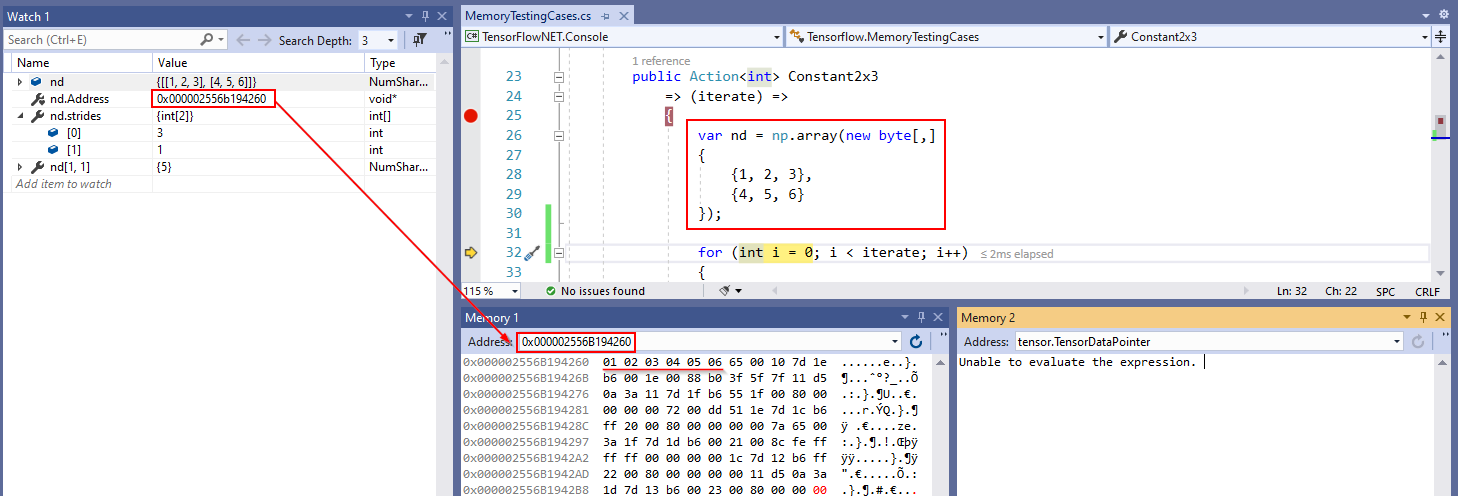# Chapter 2. Constant¶

In TensorFlow, a constant is a special Tensor that cannot be modified while the graph is running. Like in a linear model `y = ax + b`, constant `b` can be represented as a `Constant` Tensor. Since the constant is a Tensor, it also has all the data characteristics of Tensor, including:

• value: scalar value or constant list matching the data type defined in TensorFlow;
• dtype: data type;
• shape: dimensions;
• name: constant’s name;

## How to create a Constant¶

TensorFlow provides a handy function to create a Constant. In TF.NET, you can use the same function name `tf.constant` to create it. TF.NET takes the same name as python binding for the API. Naming, although this will make developers who are used to C# naming convention feel uncomfortable, but after careful consideration, I decided to give up the C# convention naming method. One of reason is for model developer, they don’t have to learn a totally new different APIs.

Initialize a scalar constant:

```var c1 = tf.constant(3); // int
var c2 = tf.constant(1.0f); // float
var c3 = tf.constant(2.0); // double
var c4 = tf.constant("Big Tree"); // string
```

Initialize a constant through ndarray:

TF.NET works very well with `NumSharp`’s `NDArray`. You can create a tensor from .NET primitive data type and NDArray as well. An `ndarray` is a (usually fixed-size) multidimensional container of items of the same type and size. The number of dimensions and items in an array is defined by its `shape`, which is a tuple of N non-negative integers that specify the sizes of each dimension.

```// dtype=int, shape=(2, 3)
var nd = np.array(new int[,]
{
{1, 2, 3},
{4, 5, 6}
});
var tensor = tf.constant(nd);
```

## Dive in Constant¶

Now let’s explore how `constant` works in `eager` mode inside the black box.

Let’s continue using the last examples, we’re going to initialize a tensor in an ndarray of `[shape(2, 3), int32]`.

### NDArray¶

The first thing we need to know is about `ndarray`’s memory model. The ndarray memory model is a very important data structure, and almost all underlying computation are inseparable from this datb a structure. One fundamental aspect of the ndarray is that an array is seen as a “chunk” of memory starting at some location. The interpretation of this memory depends on the stride information.If we take a look at the real memory allocation in Visual Studio, below diagram helps us understand the data structure more intuitively. The strides keep track the size of every single dimension, help identify the actual offset in heap memory. The formula to calculate offset is: `offset = i * strides + j * strides`.

For example: if you want to seek the value in `[1, 1]`, you just need to calculate `1 * 3 + 1 * 1 = 4`, converted to pointer is `0x000002556B194260 + 4 = 0x000002556B194264` where has a value `05`.Through the above diagram, we know how the data is stored in memory, and then we will look at how the data is transferred to `TensorFlow`.

### Tensor¶

If you don’t understand very well what `Tensor` is, you can go back to the chapter `Tensor` there is pretty much explanation if you skipped that chapter. Tensor is actually an NDArray that is with more than 2 dimensions.

TensorFlow will decide whether to copy the data or use the same pointer. Normally speaking, it’s more safe whenever you copy data for the following process, especially in interoperating between .NET runtime and C++ runtime that they all have their own garbage collection (GC) mechanism, application will crash if someone access a block of destroyed memory.### Other functions to create a Constant¶

• tf.zeros
• tf.zeros_like
• tf.ones
• tf.ones_like
• tf.fill